# Noise and SNR

Nov 19, 2020

This article demonstrates measurement of total rms integrated noise in the frequency and time domains using the Analog Discovery spectrum analyzer and scope. The noise source examined here is a fairly uniform synthesized white noise source extending from DC to about 48 kHz with a fairly sharp cutoff. It was generated by WaveLab Pro as a 24bit/96kHz wav file and output as an analog noise source using a low distortion X-Fi Elite Pro sound card. In addition, a 24bit/96kHz 1kHz test signal was similarly generated at ~ -1dBFS of the Analog Discovery input range (1.95Vrms) for an SNR demonstration.

The Right Mark Audio Analyzer traces below show the 1kHz test signal alone, with a very low noise floor of the sound card (within the 46kHz noise bandwidth) and low harmonic distortion levels and also the white noise signal (linear f scale) showing fairly uniform white noise from DC to 46kHz. These measurements were performed with a loop-back connection from the lineout --> linein of the sound card as shown: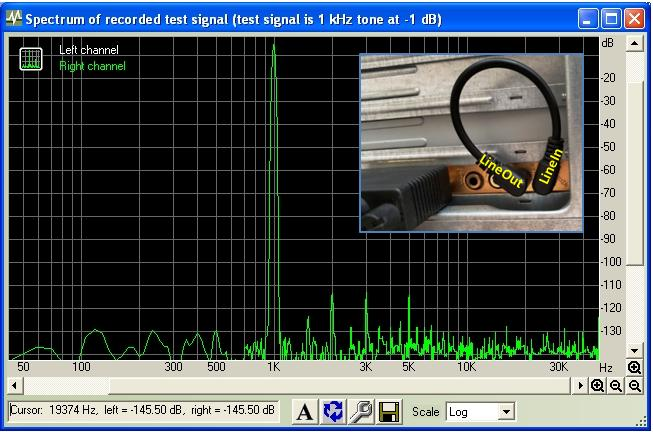Measurement of noise distributed continuously over a range of frequencies must always specify the noise measurement bandwidth. The analog bandwidth of the Analog Discovery scope is about 30 MHz (with the AD conversion at the Discovery inputs occurring at 100MHz). Internally, the effective sampling rate is usually downsized to a smaller rate and this is described by the Sampling Mode which may filter the noise measurement bandwidth. The scope operation by default uses an AVERAGE mode (averaging the N number of oversamples) which effectively changes the LPF range as the scope time-base is changed. By contrast the FFT operation by default uses the DECIMATE mode (which just takes 1 of the N number of oversamples) which does not filter. Depending on the noise range, the total noise results for the two sampling modes may be identical (if the sampled frequency range extends beyond a dominant noise range of interest).

For an FFT spectrum, the definitions of Total Noise (over some frequency range) and the SNR value derived from it are: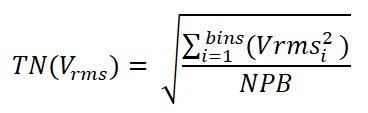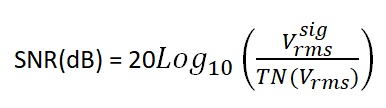where NPB is the "Noise Power Bandwidth Factor" (termed ENBW .. Effective Noise BandWidth in Waveforms) correction required to compensate for the sampling window used in the FFT. This is required as most FFT sampling window algorithms overlap adjacent sampled bins and thus the RMS summed noise voltages would overestimate the true noise in the full sampled frequency range (100kHz in this example) if not corrected for NPB. For example, the default sample window for the version of the Analog Discovery Waveforms software used here is the Flat Top algorithm which has a NPB value of 3.7706

For this study, a sampling rate of 200kHz was used, well above the 48kHz range of the white noise to be measured. This means a frequency range of the FFT from DC to 100kHz with 8192 samples (4097 bins). For the scope, this is equivalent to a time base of 4mS/div with.

The screen shots below show (1) the noise floor (TN ~ -43dBV or 6.7mVrms) of the Analog Discovery test configuration (without the input noise source signal applied); the first two scope traces show the considerable LPF filtering effect of the broadband background noise spectrum with the AVERAGE sampling mode (~ 1mVrms vs 6mVrms). (2) the test white noise source (about 18 dB higher than the Discovery-connected noise floor) alone with views in both the Spectrum Analyzer and Scope measurements, (3) the test signal alone and (4) the test signal mixed with the test white noise signal (added using WaveLab Pro and played back via the very low distortion X-Fi Elite Pro sound card line-out). The FFT measurements with the test white noise source TN (55mVrms or -25dBV) agree closely as expected with the scope rms voltage noise measurement values with the default AVERAGE sampling mode and also with the DECIMATE mode. The test signal level as measured in the FFT window (FF) and scope agree closely with Vsig = 1.73Vrms or 4.8dBV (about 1dB below the Discovery input FS value). In the combined white noise and test signal measurement, the SNR value displayed by WaveForms FFT is 16.2. The SNR value computed using the expressions above is SNR=(1.73/0.056) = 30.9. The WaveForms FFT value is low because it hasn't been corrected for the sampling window NPB factor of √3.7706 = 1.94. Applying this correction to the WaveForms FFT value gives 31.4 in good agreement with the value from the definition above with the NPB factor.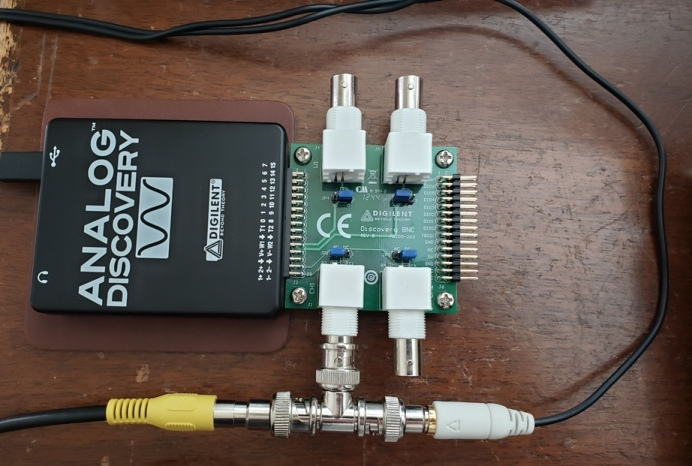The CUMULATIVE noise (from DC to any lower frequency) can be determined by exporting the captured 4k bin data covering the FFT frequency range, and using the TN expression above in an Excel chart to sum up to the frequency bin defining the range of interest. For example, in the current example, and exporting the data as Vrms, and summing over a few ranges gives:

100kHz: 56 mVrms
48kHz: 55 mVrms
24kHz: 39 mVrms
12kHz: 27 mVrms

As expected, below the noise cutoff at 48kHz, reducing the noise integration frequency range by 1/2 (48kHz --> 24kHz -->12 kHz) successively lowers the total noise by √2 ~ 0.7 as expected for a uniform white noise source.

Finally, a 5th order 20kHz LPF filter box with a rolloff of 100dB/decade or 30dB/octave was inserted between the 46kHz white noise source and the Analog Discovery to demonstrate analog filtering. The final noise traces below show the rolloff at 20kHz. The total noise (in the 100kHz Discovery frequency range setting) is reduced from 56mVrms to 37mVrms ~ 0.63 which is close to the expected reduction of √(20kHz/46kHz) = 0.66 for a flat noise spectrum. This filter also lowers the noise floor at the higher frequencies originating from the sound-card line out connection.

In most standard audio gear specification, the SNR is additionally "corrected" with an A-Weight filtering which is intended to compensate for hearing sensitivity versus frequency. This filter lowers the total noise figure and therefore raises the SNR value by about 15% or higher.

## Discovery Noise Floor: Scope Default AVERAGE Mode## Discovery Noise Floor: Scope DECIMATE Mode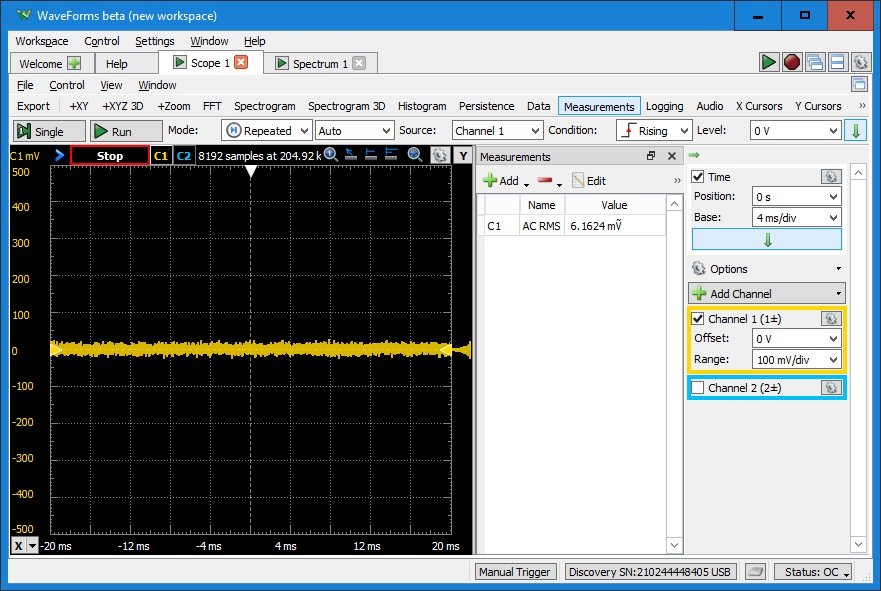## Discovery Noise Floor: FFT Default DECIMATE Mode Vrms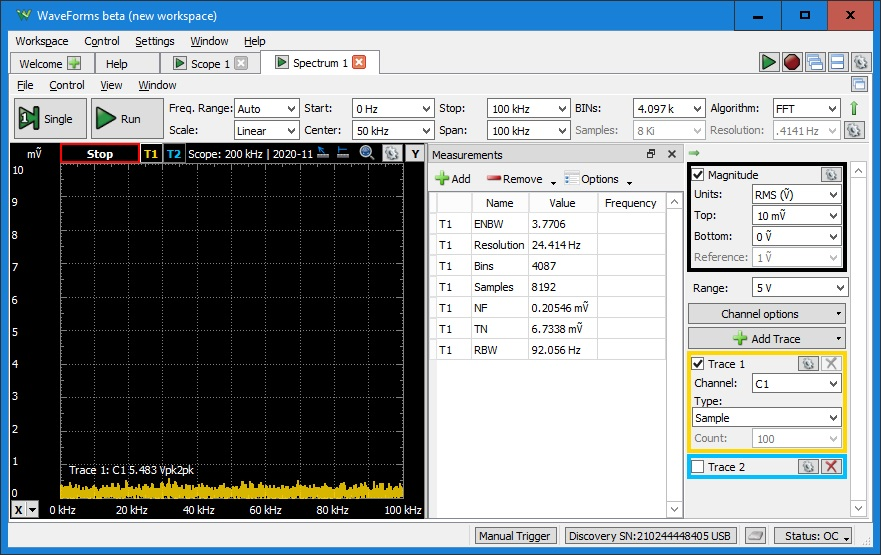## Discovery Noise Floor: FFT Default DECIMATE Mode dBV## White Noise Scope: AVERAGE mode## White Noise: FFT Vrms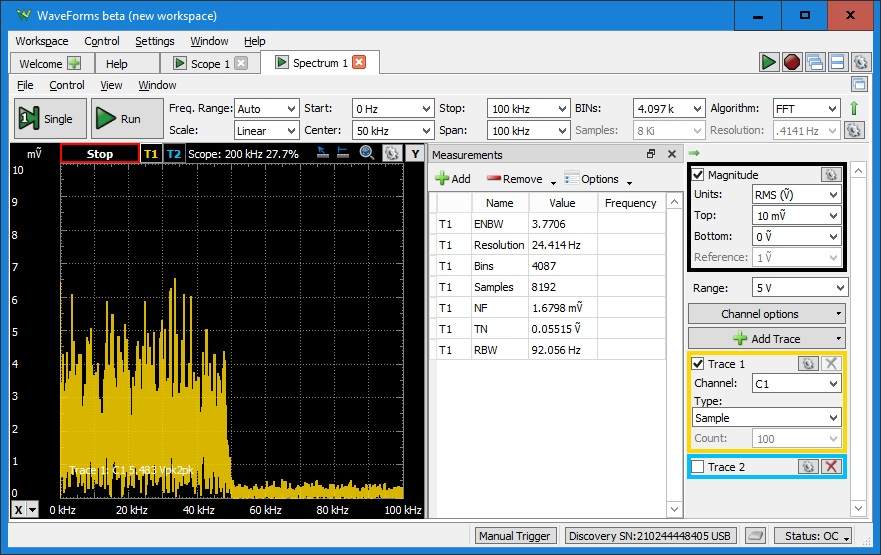## White Noise: FFT dBV## 1kHz Signal: Scope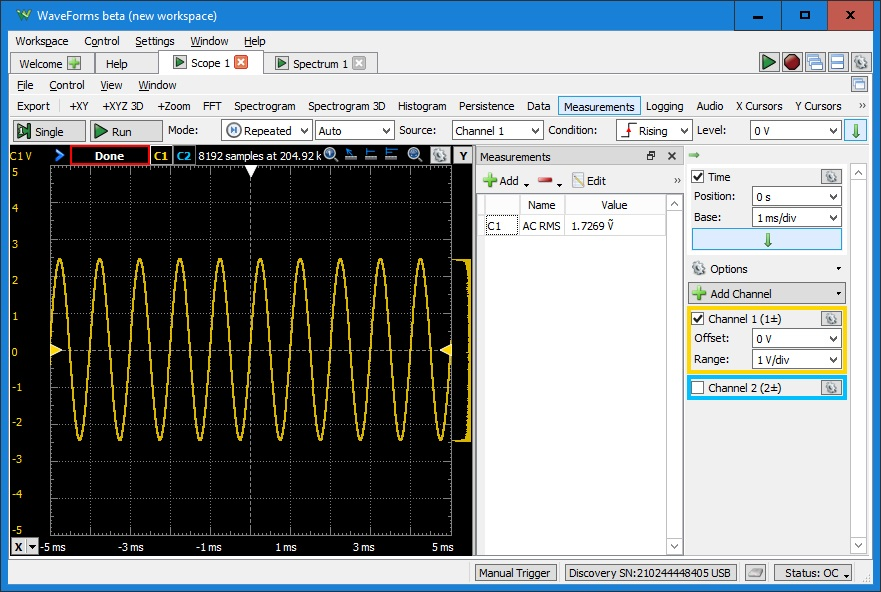## 1kHz Signal: Scope dBV## 1 kHz Signal & White Noise: Scope dBV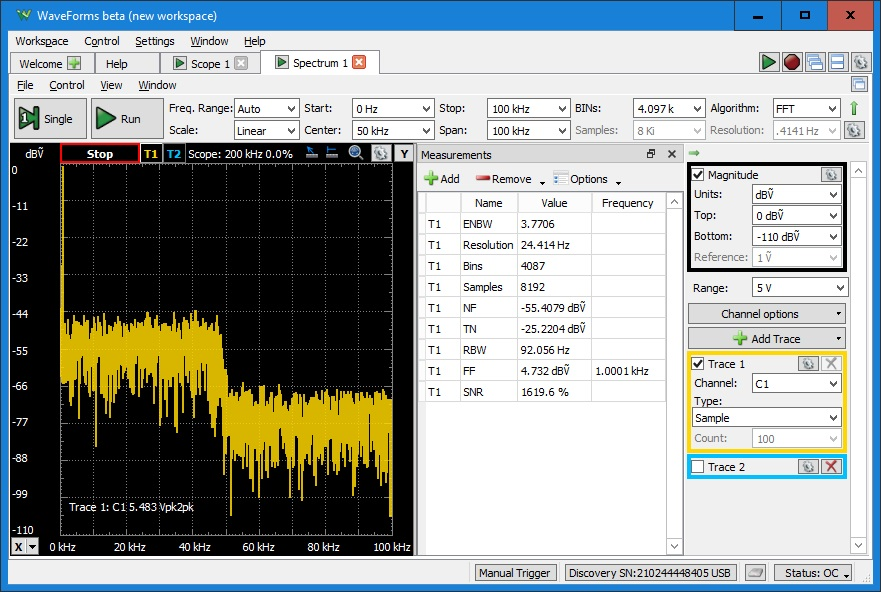## White Noise + 5th order 20kHz LPF: FFT Vrms## White Noise + 5th order 20kHz LPF: FFT dBVReferences
Audio Precision: FFT Scaling For Noise

Texas Instruments: Digital Audio Measurements (pdf)